# Physical and Chemical processes in equilibrium

Equilibrium: Physical and Chemical processes in equilibrium

A physical equilibrium is a state where the physical properties of the system remain constant with time. These properties include pressure, temperature, volume etc. For example, when water is heated, it starts vaporizing at its boiling point. At this stage, the temperature does not change until the whole liquid is turned to a gaseous state.At equilibrium, the amounts of water and its vapours remain constant but evaporation and condensation continue at the microscopic level.

There are three common types of physical equilibrium:

Solid-Liquid Equilibrium: Eg.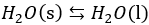, where the rate of melting is equal to the rate of freezing.

Liquid-vapourEquilibrium: Eg.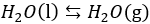, where the rate of vaporization is equal to the rate of condensation.

Solid-VaporEquilibrium: Eg.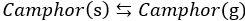, where the rate of sublimation is equal to the rate of deposition.

Solid-Solution Equilibrium Eg.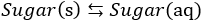, where the rate of dissolution is equal to the rate of precipitation or crystallization in a saturated solution.

Gas – Solution Equilibrium: Eg.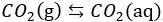, where the rate dissolution is equal to the rate of gas formation.Figure : The different phase transitions. A physical transition is always a heterogeneous change

Chemical equilibrium is a state where the concentrations of all reactants and products remain constant with time.On the molecular level, there is frantic activity. Equilibrium is not static but is a highly dynamic situation microscopically.Most chemical reactions are reversible. In a reversible reaction, the forward and backward reaction takes place under the same condition. In an irreversible reaction, the formation of products stops the reaction and the products do not react with other entities in the system.

In a chemical equilibrium, concentrations reach levels where the rate of the forward reaction equals the rate of the reverse reaction. Once the chemical reaction attains the equilibrium state, the amount of each reactant and product remains constant.Although the amounts are NOT necessarily equal, the amounts must eventually remain constant since the forward and reverse reactions are occurring simultaneously and at the same rate.

Factors that cause equilibrium in a chemical reaction are:

1. The tendency for a reaction to move towards minimum energy state.
2. The tendency for a reaction to move towards maximum entropy state.Figure : Change in reaction rate with time.

In a system at equilibrium, both the forward and reverse reactions are being carried out in a dynamic state; as a result, we write its equation with a double arrow. Consider an equilibrium mixture in a closed vessel that reacts as the following equation: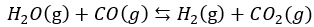In this case, when more steam is added to the reaction chamber, the concentrations of each product increases while the concentration of CO decreases. Here, the concentration of water will become higher than the original equilibrium concentration, but lower than the initial total amount. Alternatively, when more hydrogen gas is added to the reaction chamber, the concentrations of water and CO will increase. The concentration of carbon dioxide decreases and the concentration of hydrogen is higher than the original equilibrium concentration, but lower than the initial total amount.